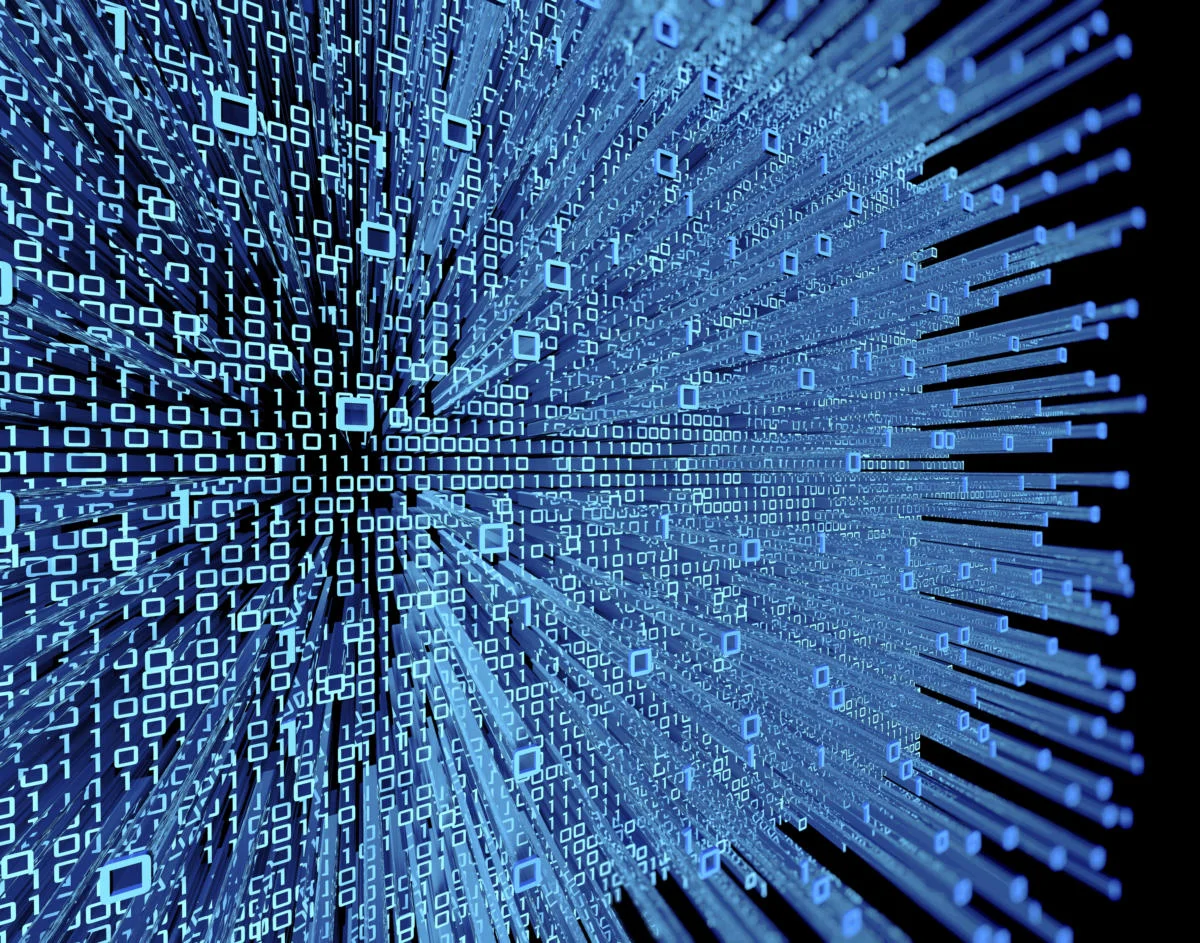Machine Learning Algorithms Explained, Machine learning and deep learning have been widely embraced, and even more widely misunderstood. In this article, I’d like to step back and explain both machine learning and deep learning in basic terms, discuss some of the most common machine learning algorithms, and explain how those algorithms relate to the other pieces of the puzzle of creating predictive models from historical data.

## What are machine learning algorithms?

Recall that machine learning is a class of methods for automatically creating predictive models from data. Machine learning algorithms are the engines of machine learning, meaning it is the algorithms that turn a data set into a model. Which kind of algorithm works best (supervised, unsupervised, classification, regression, etc.) depends on the kind of problem you’re solving, the computing resources available, and the nature of the data.

## How machine learning works

Ordinary programming algorithms tell the computer what to do in a straightforward way. For example, sorting algorithms turn unordered data into data ordered by some criteria, often the numeric or alphabetical order of one or more fields in the data.

Linear regression algorithms fit a straight line, or another function that is linear in its parameters such as a polynomial, to numeric data, typically by performing matrix inversions to minimize the squared error between the line and the data. Squared error is used as the metric because you don’t care whether the regression line is above or below the data points; you only care about the distance between the line and the points.

Nonlinear regression algorithms, which fit curves that are not linear in their parameters to data, are a little more complicated, because, unlike linear regression problems, they can’t be solved with a deterministic method. Instead, the nonlinear regression algorithms implement some kind of iterative minimization process, often some variation on the method of steepest descent.

Steepest descent basically computes the squared error and its gradient at the current parameter values, picks a step size (aka learning rate), follows the direction of the gradient “down the hill,” and then recomputes the squared error and its gradient at the new parameter values. Eventually, with luck, the process converges. The variants on steepest descent try to improve the convergence properties.

Machine learning algorithms are even less straightforward than nonlinear regression, partly because machine learning dispenses with the constraint of fitting to a specific mathematical function, such as a polynomial. There are two major categories of problems that are often solved by machine learning: regression and classification. Regression is for numeric data (e.g. What is the likely income for someone with a given address and profession?) and classification is for non-numeric data (e.g. Will the applicant default on this loan?).

Prediction problems (e.g. What will the opening price be for Microsoft shares tomorrow?) are a subset of regression problems for time series data. Classification problems are sometimes divided into binary (yes or no) and multi-category problems (animal, vegetable, or mineral).